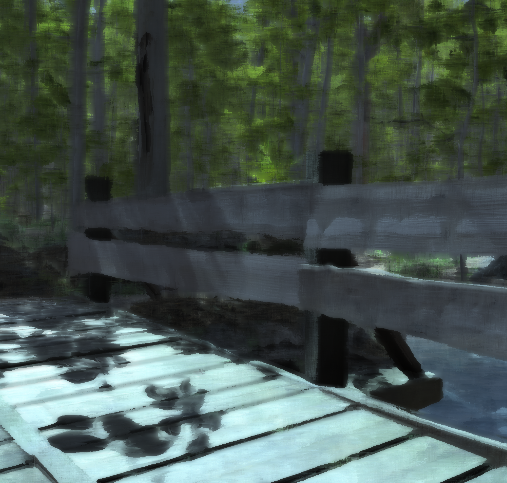Hi All I am trying to port an image Effect Shader to Compute Shader because I want to learn compute shaders. I chose to port Kuwahara Filter because I am generally interested in image filtering. Below is my port which give a black screen. Any help will be appreciated as I am very new to this and there isn’t much info on the subject around. The original image effect shader is in the link above.

``````//
#pragma kernel CSMain
#include "UnityCG.cginc"

// Create a RenderTexture with enableRandomWrite flag and set it
// with cs.SetTexture

Texture2D<float4> Source;
RWTexture2D<float4> Destination;
SamplerState samplerSource;

{

float3 mean = {
{ 0, 0, 0 },
{ 0, 0, 0 },
{ 0, 0, 0 },
{ 0, 0, 0 }
};

float3 sigma = {
{ 0, 0, 0 },
{ 0, 0, 0 },
{ 0, 0, 0 },
{ 0, 0, 0 }
};

float2 pos;
float3 col;
uint width;
uint height;
uint levels;
Source.GetDimensions(0, width, height, levels);

float widthf = (float)width;
float heightf = (float)height;

for (int k = 0; k < 4; k++) {
for (int i = 0; i <= Radius; i++) {
for (int j = 0; j <= Radius; j++) {
pos = float2(i, j) + start[k];
col = Source.SampleLevel(samplerSource, float2(pos.x * 1.0/width, pos.y * 1.0/height), 0);
mean[k] += col;
sigma[k] += col * col;
}
}
}

float sigma2;

float n = pow(Radius + 1, 2);
float4 color = Source[id.xy];
float min = 1;

for (int l = 0; l < 4; l++) {
mean[l] /= n;
sigma[l] = abs(sigma[l] / n - mean[l] * mean[l]);
sigma2 = sigma[l].r + sigma[l].g + sigma[l].b;

if (sigma2 < min) {
min = sigma2;
color.rgb = mean[l].rgb;
}
}

Destination[id.xy] = color;

}
``````

bit of a late reply, but this works for me:

``````#pragma kernel CSMain
#include "UnityCG.cginc"

Texture2D<float4> source;
RWTexture2D<float4> output;

SamplerState sampler_source;

{
float3 mean = {
{ 0, 0, 0 },
{ 0, 0, 0 },
{ 0, 0, 0 },
{ 0, 0, 0 }
};

float3 sigma = {
{ 0, 0, 0 },
{ 0, 0, 0 },
{ 0, 0, 0 },
{ 0, 0, 0 }
};

float2 start = { { -_Radius * .5, -_Radius * .5},{ -_Radius * .5, 0 },{ 0, -_Radius * .5},{ 0, 0 } };

float2 pos;
float3 col;

for (int k = 0; k < 4; k++) {
for (int i = 0; i <= _Radius; i++) {
for (int j = 0; j <= _Radius; j++) {
pos = float2(i, j) + start[k];
col = source[id.xy + uint2(pos.x, pos.y)];
mean[k] += col;
sigma[k] += col * col;
}
}
}

float sigma2;

float n = pow(_Radius + 1, 2);
float4 color = source[id.xy];
float min = 1;

for (int l = 0; l < 4; l++) {
mean[l] /= n;
sigma[l] = abs(sigma[l] / n - mean[l] * mean[l]);
sigma2 = sigma[l].r + sigma[l].g + sigma[l].b;

if (sigma2 < min) {
min = sigma2;
color.rgb = mean[l].rgb;
}
}

output[id.xy] = color;

}
``````Not too lateThanks! I will check it out. Also, you another new thing that you might want to Check out is a library called ComputeSharp (not unity related)